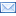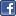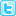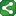## Wednesday, August 27, 2008

### CLASS Statement

When should I put a variable in the CLASS statement? What does the CLASS statement do?

The CLASS statement is used to indicate which variables in the model are categorical variables. In most modeling procedures, such a variable is then treated as a nominal (unordered) categorical predictor variable. A set of numeric indicator ("dummy") variables is created internally to represent the levels of the variable. Because the indicator variables are used for fitting the model, the original variable does not need to be numeric. The resulting model has multiple parameter estimates (one for each indicator variable). Each parameter compares one level of the predictor with a reference level, typically the last level in sorted order. A joint test of all the estimated parameters for the predictor is a test for any differences among the levels and is therefore a test of the predictor's overall effect.

In contrast, a variable name that appears in the MODEL statement but not in the CLASS statement is treated as a continuous predictor variable. The variable itself is used in fitting the model. Therefore, the variable must be a numeric SAS variable and should be continuous or at least be ordered with assigned numeric scores. The resulting model typically has one parameter estimate (there might be more for models with multiple response variables or functions) that estimates the linear effect of the predictor.

Note that if the predictor was unordered, it would not be useful to test for its "linear" effect because you cannot talk about the effect of "increasing" an unordered variable. So, all nominal, categorical variables should be listed in the CLASS statement. On the other hand, you might choose to ignore the ordering in a continuous predictor variable and treat it as a nominal predictor by specifying it in the CLASS statement. But remember that a parameter will be added to the model for each additional level of the variable and this could result in a very large model if the variable has many distinct values in the data set.

Some procedures (such as PROC LOGISTIC) offer many options in the CLASS statement that enable you to designate how the internally generated variables are coded. Each coding method imposes a different interpretation on the estimated parameters. For instance, the GLM (or indicator or dummy) coding method that was mentioned earlier creates parameter estimates that compare the effect of each level to the effect of the reference level. Another coding method for nominal predictors is effects coding, which results in parameter estimates that compare the effect of each level to the average effect of all the levels. There is a coding method appropriate for variables that are ordinal but with unknown spacing between the levels. And there is a coding method for continuous variables that decomposes the variable's effect into linear, quadratic, cubic, and other components.

source:
www.support.sas.comShareThis